Related Articles

# Mathematical Operations on Arrays in Julia

• Last Updated : 26 May, 2020

With the help of Mathematical Operations, we can perform addition, subtraction, multiplication, division, and many more to compute the result between two matrices or arrays. Mathematical operations are a very crucial part of any high-level programming language. In the world of Julia to compete with languages like python and java, Julia is also enabled with the same functionality but have different syntax.

Mathematical Operations :

[1 2 3] + [4 5 10] = [5 7 13]

Subtration: This operation helps to subtract two arrays.
[1 2 3] – [4 5 10] = [-3 -3 -7]

Multiplication: This operation helps to multiply two arrays.
[1 2 3] * [4; 5; 10] = 

Division: This operation helps to divide two arrays.
[1 2 3] / [4; 5; 10] = [3.1428…]

We can add two arrays with the help of + operator.

Example of 1D array :

 `# Define and declare the 1D arrays``A ``=` `[``1` `2` `3``] ``# Shape 1X3``B ``=` `[``4` `5` `10``] ``# Shape 1X3`` ` `# Adding two arrays``gfg ``=` `A ``+` `B``print``(gfg)`

Output: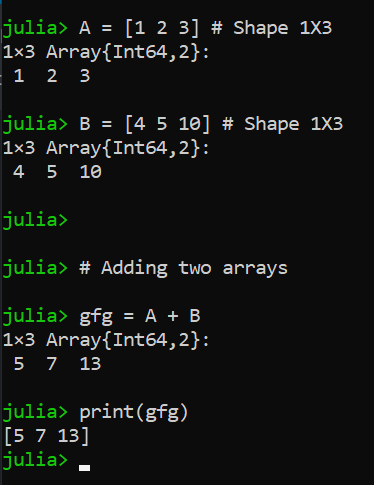Example of 2D array:

 `# Define and declare the 2D arrays``A ``=` `[``1` `2``; ``-``1` `-``2``] ``# Shape 2X2``B ``=` `[``4` `5``; ``10` `12``] ``# Shape 2X2`` ` `# Adding two arrays``gfg ``=` `A ``+` `B``print``(gfg)`

Output: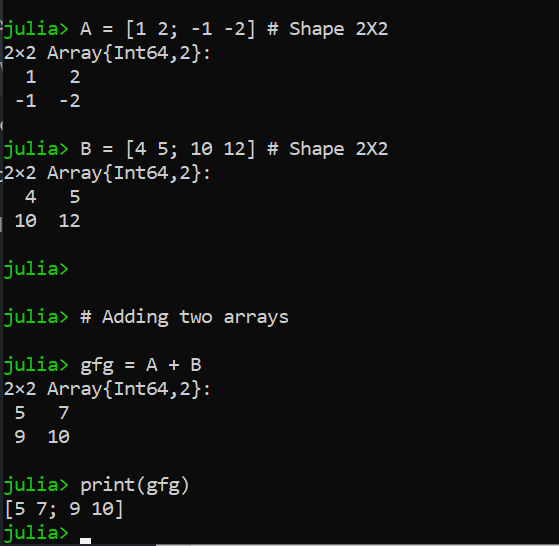Example of 3D array:

 `# Define and declare the 3D arrays``A ``=` `cat([``1` `2` `3``], [``-``1` `-``2` `-``3``], [``2` `1` `4``], dims``=``3``) ``# Shape 3X3``B ``=` `cat([``4` `5` `2``], [``10` `12` `-``5``], [``-``1` `2` `1``], dims``=``3``) ``# Shape 3X3`` ` `# Adding two arrays``gfg ``=` `A ``+` `B``print``(gfg)`

Output: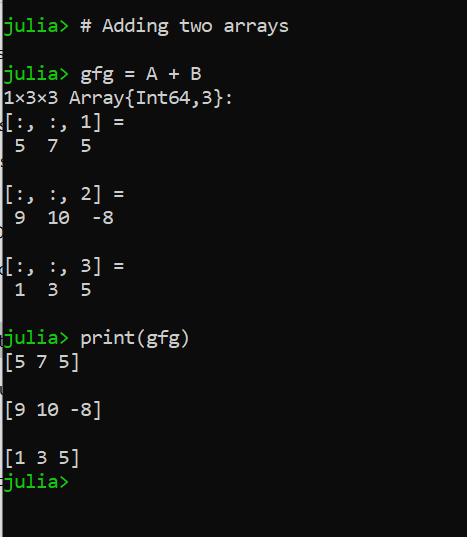#### Subtraction operation

We can subtract two arrays with the help of operator.

Example of 1D array:

 `# Define and declare the 1D arrays``A ``=` `[``1` `2` `3``] ``# Shape 1X3``B ``=` `[``4` `5` `10``] ``# Shape 1X3`` ` `# Subtracting two arrays``gfg ``=` `A ``-` `B``print``(gfg)`

Output: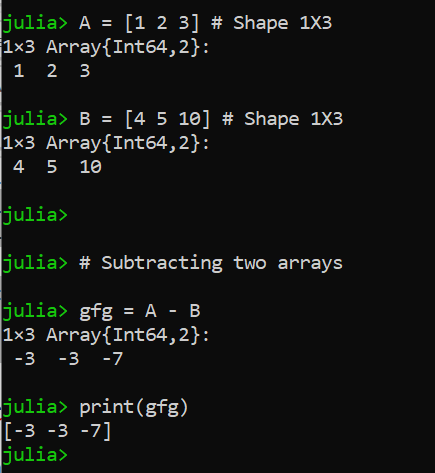Example of 2D array:

 `# Define and declare the 2D arrays``A ``=` `[``1` `2``; ``-``1` `-``2``] ``# Shape 2X2``B ``=` `[``4` `5``; ``10` `12``] ``# Shape 2X2`` ` `# Subtracting two arrays``gfg ``=` `A ``-` `B``print``(gfg)`

Output: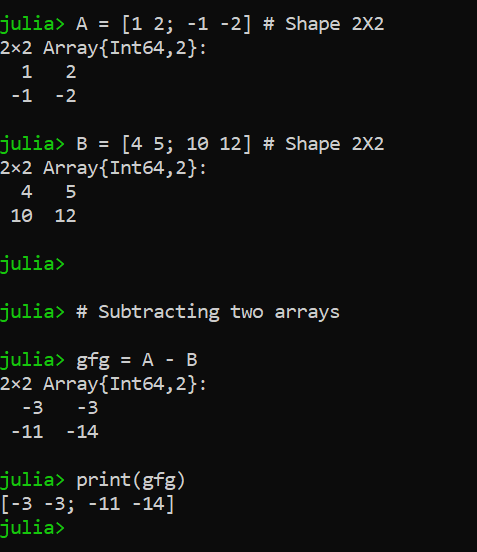Example of 3D array:

 `# Define and declare the 3D arrays``A ``=` `cat([``1` `2` `3``], [``-``1` `-``2` `-``3``], [``2` `1` `4``], dims``=``3``) ``# Shape 3X3``B ``=` `cat([``4` `5` `2``], [``10` `12` `-``5``], [``-``1` `2` `1``], dims``=``3``) ``# Shape 3X3`` ` `# Subtracting two arrays``gfg ``=` `A ``-` `B``print``(gfg)`

Output: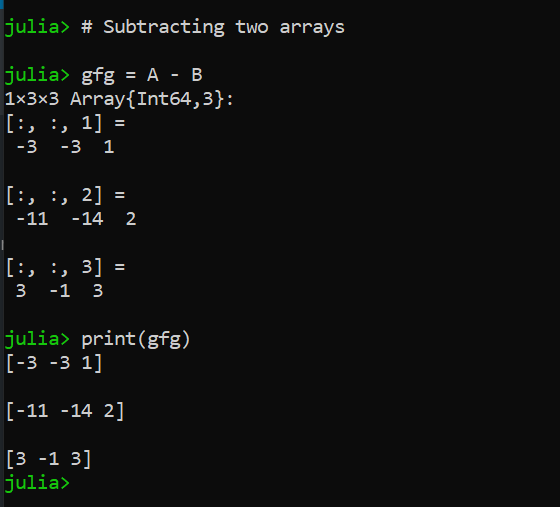#### Multiplication operation

We can multiply two arrays with the help of * operator.

Example of 1D array:

 `# Define and declare the 1D arrays``A ``=` `[``1` `2` `3``] ``# Shape 1X3``B ``=` `[``4``; ``5``; ``10``] ``# Shape 3X1`` ` `# Multiplying two arrays``gfg ``=` `A ``*` `B``print``(gfg)`

Output: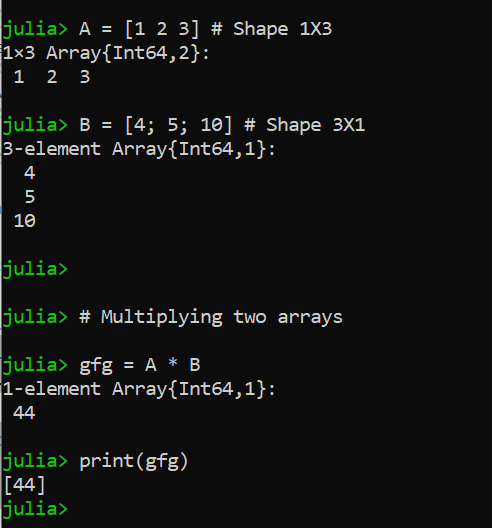Example of 2D array:

 `# Define and declare the 2D arrays``A ``=` `[``1` `2``; ``-``1` `-``2``] ``# Shape 2X2``B ``=` `[``4` `5``; ``10` `12``] ``# Shape 2X2`` ` `# Multiplying two arrays``gfg ``=` `A ``*` `B``print``(gfg)`

Output: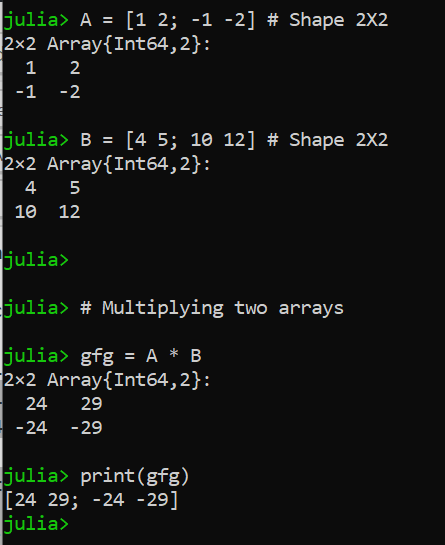Example of 3D array:

 `# Define and declare the 3D arrays``A ``=` `cat([``1` `2` `3``], [``-``1` `-``2` `-``3``], [``2` `1` `4``], dims``=``3``) ``# Shape 3X3``B ``=` `cat([``4` `5` `2``], [``10` `12` `-``5``], [``-``1` `2` `1``], dims``=``3``) ``# Shape 3X3`` ` `# Multiplying two arrays``gfg ``=` `A ``*` `B``print``(gfg)`

Output: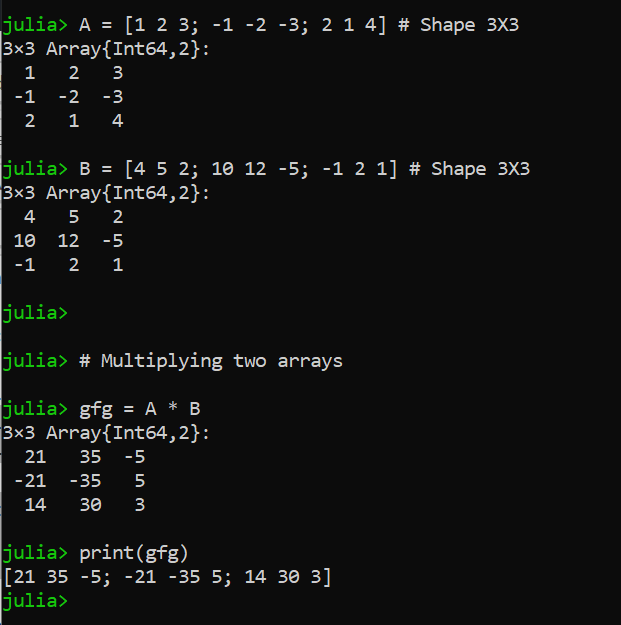#### Division operation

We can division two arrays with the help of / operator.

Example of 1D array:

 `# Define and declare the 1D arrays``B ``=` `[``1` `2` `3``] ``# Shape 1X3``A ``=` `[``4` `5` `10``] ``# Shape 1X3`` ` `# Dividing two arrays``gfg ``=` `A ``/` `B``print``(gfg)`

Output: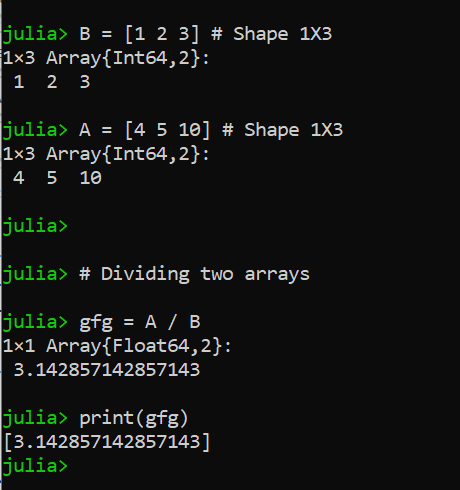Example of 2D array:

 `# Define and declare the 2D arrays``B ``=` `[``1` `2``; ``1` `2``] ``# Shape 2X2``A ``=` `[``4` `5``; ``10` `12``] ``# Shape 2X2`` ` `# Dividing two arrays``gfg ``=` `B ``/` `A``print``(gfg)`

Output: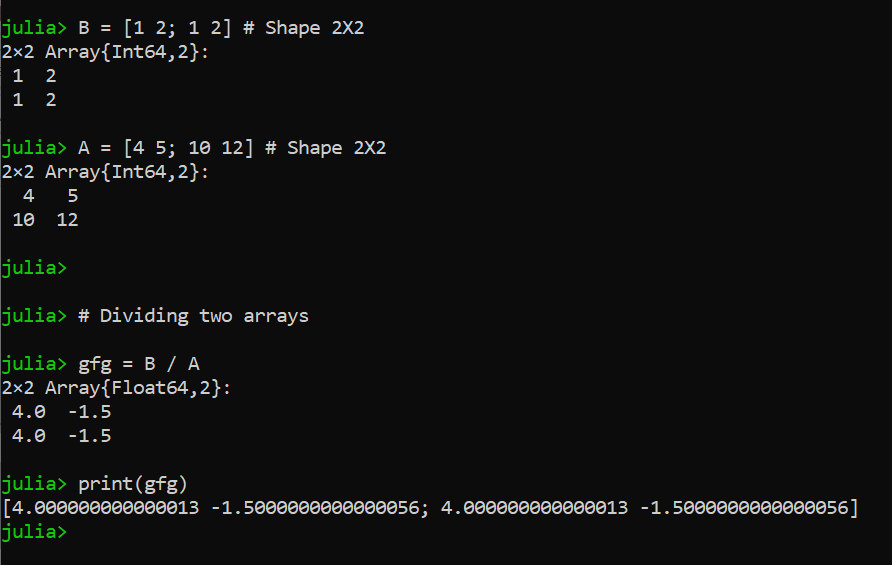Example of 3D array:

 `# Define and declare the 3D arrays``A ``=` `cat([``1` `2` `3``], [``-``1` `-``2` `-``3``], [``2` `1` `4``], dims``=``3``) ``# Shape 3X3``B ``=` `cat([``4` `5` `2``], [``10` `12` `-``5``], [``-``1` `2` `1``], dims``=``3``) ``# Shape 3X3`` ` `# Dividing two arrays``gfg ``=` `B ``/` `A``print``(gfg)`

Output: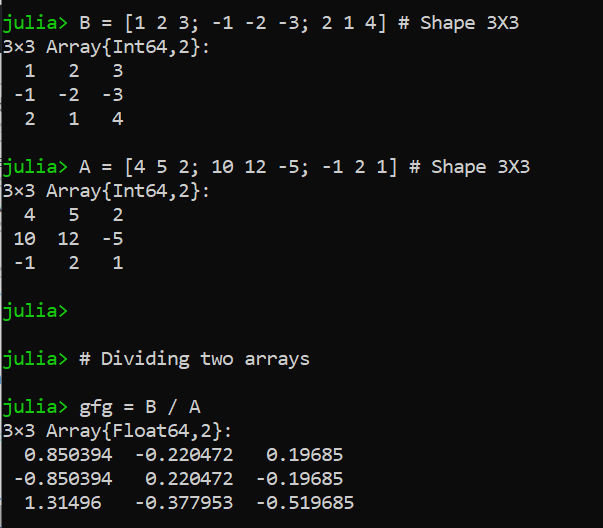My Personal Notes arrow_drop_up